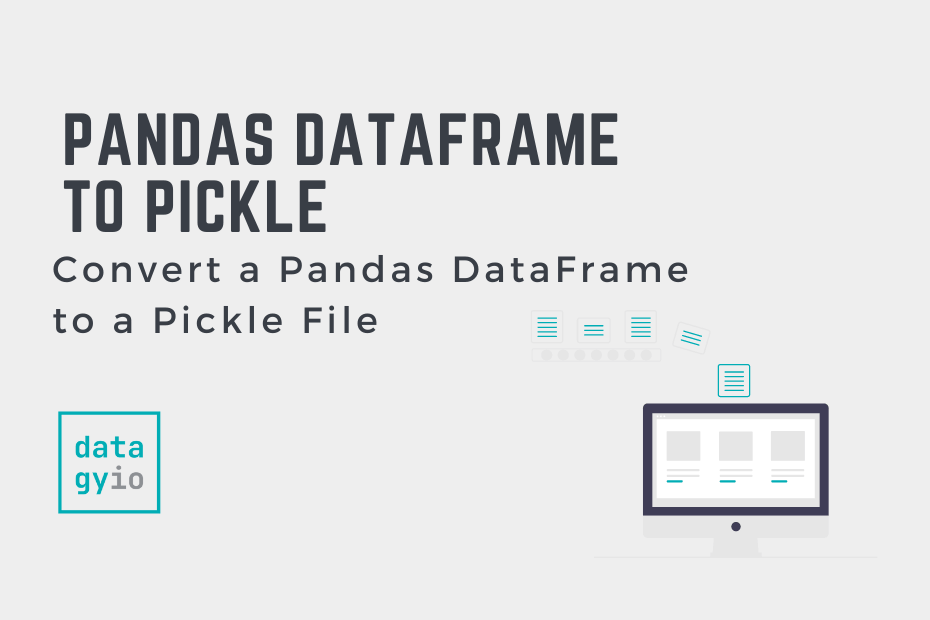# Convert a Pandas DataFrame to a Pickle FilePickle files are serialized data structures that allow you to maintain data state across sessions. Pickle files are incredibly common in data science. In this tutorial, you’ll learn how to serialize a Pandas DataFrame to a Pickle file. This is an important function to understand, given the prevalence of pickle files in data science workflows.

By the end of this tutorial, you’ll have learned:

• How to use the Pandas `.to_pickle()` method
• How to serialize a Pandas DataFrame and a Pandas Series to pickle files

## Understanding the Pandas to_pickle Method

Before diving into using the Pandas `.to_pickle()` method, let’s take a look at how the function is made up. This can give a good sense of how you can customize and use the function to meet your needs.

``````# Understanding the Pandas .to_pickle() Method
import pandas as pd
df = pd.DataFrame()
df.to_pickle(path, compression='infer', protocol=5, storage_options=None)``````

The table below breaks down the different parameters and default arguments of the method:

Now that you have a strong understanding of the `.to_pickle()` method, let’s load a sample Pandas DataFrame to follow along the tutorial with.

In this section, you’ll create a sample Pandas DataFrame to follow along with. If you’re working with your own data, your results will, of course, reflect that data. To follow along line-by-line, simply copy and paste the code form the code block below into your code editor of choice:

``````# Loading a Sample Pandas DataFrame
import pandas as pd
df = pd.DataFrame({
'Name': ['Nik', 'Katie', 'Evan'],
'Age': [34, 33, 27],
'Location': ['Toronto', 'NYC', 'Atlanta']
})

print(df)

# Returns:
#     Name  Age Location
# 0    Nik   34  Toronto
# 1  Katie   33      NYC
# 2   Evan   27  Atlanta``````

We can see that our DataFrame has three columns and three records. Now that we have a DataFrame, let’s learn how to convert it into a serialized Pickle file.

## Convert a Pandas DataFrame to a Pickle File

The Pandas `.to_pickle()` method has only one required argument, the path to which to save the serialized file. Because the `path=` parameter is the first parameter positionally, you can simply pass in a string representing the path to which you want to save the file.

Let’s see how we can serialize our Pandas DataFrame to a Pickle file:

``````# Serializing a Pandas DataFrame to a Pickle File
import pandas as pd
df = pd.DataFrame({
'Name': ['Nik', 'Katie', 'Evan'],
'Age': [34, 33, 27],
'Location': ['Toronto', 'NYC', 'Atlanta']
})

df.to_pickle('pickle.pkl')``````

When we serialize our DataFrame, we maintain its state. This means that any transformations we previously applied to the data will be retained. This can be particularly helpful when working with large data models that need to be referenced.

We can read part of the serialized file using a context manager and the `.read()` method.

``````# Reading Part of the Serialized File
with open('pickle.pkl', 'rb') as file:

# Returns:
# b'\x80\x05\x953\x03\x00\x00\x00\x00\x00\x00\x8c\x11pandas.core.frame\x94\x8c\tDataFrame\x94\x93\x94)\x81\x94}\x94'``````

In the code block above, we open the file using a context manager. We then use the `.read()` method to read the first fifty characters of the serialized file.

In the following section, you’ll learn how to serialize a single Pandas column (or, rather, a Pandas Series) to a pickle file.

## Adding Compression When Pickling a Pandas DataFrame

We can apply additional compression when serializing Pandas DataFrames to pickle files. Pandas supports a wide variety of different compression formats. The following formats are available: ‘.gz’, ‘.bz2’, ‘.zip’, ‘.xz’, ‘.zst’, ‘.tar’, ‘.tar.gz’, ‘.tar.xz’ or ‘.tar.bz2’.

In order to apply additional compression, we can specify the type of compression we want to use in the `compression=` parameter. Let’s see how we can add zip compression to our file:

``````# Adding zip Compression to a Pickle File
import pandas as pd
df = pd.DataFrame({
'Name': ['Nik', 'Katie', 'Evan'],
'Age': [34, 33, 27],
'Location': ['Toronto', 'NYC', 'Atlanta']
})

df.to_pickle(path='pickle_compressed.zip', compression='zip')``````

It’s important to note that when you wish to read the pickle file in the future, you will need to specify the compression method in reading the file.

## Convert a Pandas Series (Column) to a Pickle File

We can also serialize a single Pandas DataFrame column (Pandas Series) to a pickle file. This can be done by applying the `.to_pickle()` method to the Series. The process works in the same way as serializing an entire DataFrame to a pickle file.

Let’s see how we can convert a Pandas Series to a pickle file:

``````# Serializing a Single Pandas DataFrame Column
import pandas as pd
df = pd.DataFrame({
'Name': ['Nik', 'Katie', 'Evan'],
'Age': [34, 33, 27],
'Location': ['Toronto', 'NYC', 'Atlanta']
})

df['Age'].to_pickle('pickle_series.pkl')``````

## Conclusion

In this tutorial, you learned how to save a Pandas DataFrame to a serialized pickle file. You first learned about the Pandas `.to_pickle()` method and its various parameters. You then learned how to save a Pandas DataFrame to a pickle file. Following that, you learned how to apply additional compression to the file. Finally, you learned how to serialize a single Pandas Series.